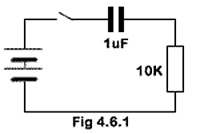# DC Transients Quiz

Try our quiz, based on the information you can find in Module 4. Hopefully it won't be too difficult. Submit your answers to see how well you did. If you got any answers wrong, you´ll be told where to find the right answer, so you can learn more about transient events before you have another try.

### 1.When the switch in Fig. 4.6.1 is closed, how long will it take for the capacitor voltage to reach its steady state value?

### 2.When the switch in Fig. 4.6.2 is closed, how long will it take for the capacitor voltage to rise to 6.3 volts?

### 3.

A CR circuit connected to a DC supply will, for a time after a voltage is applied to it, behave as though the capacitors were very ________ value resistances, but these effects will disappear after a time approximately equal to _______ time constant(s). The missing words in this sentence are:

### 4.Refer to Fig. 4.6.3: What will be the approximate voltage across the inductor 100ns after the switch is closed?

### 5.

Calculate the time constant of a circuit consisting of a 15mH inductor and a 470Kohm resistor.

### 6.

If a simple LR circuit consisting of a 20Ω resistor in series with a 100mH inductor is connected to a 10V supply, from which it draws a current of 500mA after it has reached its steady state. How long after switch on, will the current take to reach 316mA?

### 7.

An ideal circuit is a useful theoretical tool because:

### 8.

In the formula I = E/(R+r) the quantity r is added to compensate for:

### 9.

The back EMF produced as an inductor charges up is proportional to:

### 10.

The back e.m.f produced when an inductor is first connected to a DC supply is..

Top of Page.>